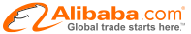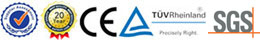More and more automatic in Cutting!
cuttingmachine-hg1
0086 15918086545
Home > Resources > F.A.Q > How to determine the pressure of the cutting machine?

Resources

How to determine the pressure of the cutting machine?

How to determine the pressure of the cutting machine?

The cutting machine pressure calculation method is:
system pressure by cylinder piston area; this formula we will calculate, the key is not aware of the specific value so can not be calculated.
But if you are careful to find the hydraulic pump is rated pressure rating on the nameplate, remember the value, and then out of the cylinder diameter; system pressure multiplied by the cylinder piston area multiplied by the number of cylinders, so that we can roughly calculate the machine pressure.

The above is the calculation method, now how we see the specific pressure cutting machine:

1, directly in the hydraulic system and then connected to the pressure gauge, pressure value; if this value is close to the rated value of the hydraulic pump, then the machine pressure is normal. If the value difference is too large, then there is a leak in the hydraulic circuit or hydraulic pump pressure decay. Now the value of the hydraulic pump rated percentage, you can calculate the cutting machine now the actual pressure.

2, take the pressure detector directly on the bench to get the pressure reading, this is very simple, the instrument has measured the value converted to tons, but the instrument is more expensive, the general manufacturers will not buy a back to use.

3, based on experience, listen to the sound, to see the movement to estimate, this must have some basic skills or long time exposure to the machine's ability to see it.

From
Honggang Cutting Machine co.ltd